# Black Hole

A black hole is an object that meets the conditions that even light cannot escape from it. The idea of a black hole arises from the concepts of Einstein's general relativity, particularly the curvature of space-time by the gravity of a massive object. The fact that light follows this curvature suggests the possibility of a curvature so extreme that light cannot escape the vicinity of the object. The gravity of a massive object interacts with photons to produce a gravitational red shift, representing a decrease in photon energy with increasing radial distance. If the photon frequency and energy are red-shifted to zero, this is another way of saying that the photon cannot escape. The red shift to zero is associated with a radius, the Schwarzschild radius. Using the existence of a Schwarzschild radius to characterize a black hole, it becomes evident that a black hole with larger mass will have a larger Schwarzschild radius so that black holes could conceivably exist with any mass provided that mass could be contained in a sufficiently small radius. The development that describes the gravitational red shift also points to gravitational time dilation, the fact that being closer to a gravitational mass will progressively slow down the progress of time. As an object approaches the Schwarzschild radius, the progress of time approaches zero so that time stands still. This radius is commonly called the "event horizon" since no information is available past this radius. If a massive object is contracting, there are certain processes which tend to halt the contraction, such as electron degeneracy which may stop the contraction to form a white dwarf. If the mass is too great for a white dwarf, neutron degeneracy may halt it to form a neutron star. But if the mass is greater than two to three solar masses there is no known mechanism to halt further collapse and it is presumed not only that a black hole will result from the collapse, but that the collapse will continue toward zero spatial extent, a singularity.

Index

General relativity ideas

Black hole concepts

 HyperPhysics***** Relativity R Nave
Go Back

# Gravity and the Photon

The relativistic energy expression attributes a mass to any energetic particle, and if applied to the photon with its zero rest mass: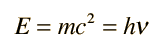The effective gravitational potential energy* is thenIf an emitted photon of frequency υ0 moves relative to the gravitational mass, it will have a measurably different frequency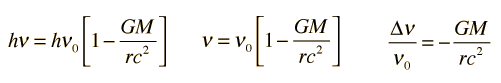Since it is reduced in frequency, this is called the gravitational red shift or the Einstein red shift. The above expression is an approximation for weak gravity fields, which can be applied to small laboratory displacements on the Earth like the Harvard Tower experiment, but can be generalized for gravitational fields of any strength.

*The idea of "gravitational potential energy" for a photon must be brought into consistency with general relativity, which would attribute the gravity effect to the local curvature of space-time. So any "effective mass" for the photon is subject to the same difficulties as the "relativistic mass" for a high speed particle.

 Escape energy for a photon
Index

General relativity ideas

 HyperPhysics***** Relativity R Nave
Go Back

# Escape Energy for Photon

While the idea of an effective gravitational potential energy* for the photon is of some usefulness in weak gravitational fields or small displacements near the Earth, it is usually more useful to work with the general expression for the gravitational red shift. Presuming that a photon is emitted at radius r0 near a gravitational mass M and is measured at a great distance from the mass, the observed frequency is given by the relationship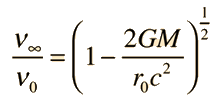If the photon frequency and energy are essentially zero at the great distance of observation, then the expression on the right has approached zero and we have the condition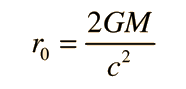Note that this condition is independent of the frequency, and for a given mass M establishes a critical radius called the Schwarzschild radius at which photons cannot escape from the mass M. This is not a derivation of the Schwarzschild radius, which comes from the Schwarzschild metric, but shows the correlation with gravitational redshift.

It is interesting historically that the Schwarzschild radius expression is coincidently equal to the gravitational escape velocity expression for velocity v=c.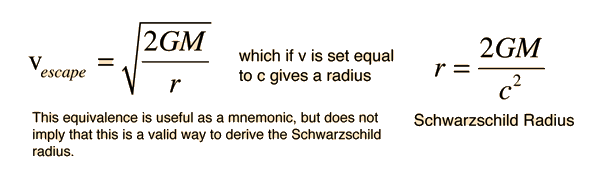In 1783 John Michell (1724-1793), an English clergyman and amateur astronomer considered the implications of Newton's corpuscular theory of light. Considering that such a stream of particles should be affected by gravity, he speculated that for a sufficiently massive body, light would not be able to escape.

*The idea of "gravitational potential energy" for a photon must be brought into consistency with general relativity, which would attribute the gravity effect to the local curvature of space-time. So any "effective mass" for the photon is subject to the same difficulties as the "relativistic mass" for a high speed particle.